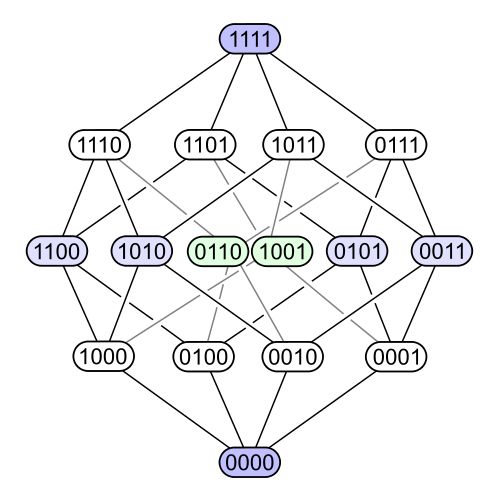#### Howdy, Stranger!

It looks like you're new here. If you want to get involved, click one of these buttons!

Options

# Exercise 108 - Chapter 1

edited June 2018

Draw the Hasse diagram for the preorder $$\mathbf{Rel}( \{ 1, 2 \} )$$ of all binary relations on the set $$\{ 1, 2 \}$$.

• Options
1.
edited April 2018

Is this what is intended?Comment Source:Is this what is intended? ![Hasse](https://docs.google.com/drawings/d/e/2PACX-1vTCgcacsG5ZRLBZqU1ZDLiM4Swx0ifCHDRRLS_GR8GN-jplFAjS_sAcNuyMrytKFgwqQplizbFjqHew/pub?w=746&h=375)
• Options
2.

Fredrick: This is my understanding of the question and how I have the answer written in my notebook. It seems like it would be the same Hasse diagram as for the powerset of a set of 4 elements. Since with $$S=\{1,2\}$$, $$S\times S=\{(1,1),(1,2),(2,1),(2,2)\}$$.

Comment Source:Fredrick: This is my understanding of the question and how I have the answer written in my notebook. It seems like it would be the same Hasse diagram as for the powerset of a set of 4 elements. Since with \$$S=\\{1,2\\}\$$, \$$S\times S=\\{(1,1),(1,2),(2,1),(2,2)\\}\$$.
• Options
3.

@JaredSummers I guess the point was noticing that $$S \times S$$ forms a set of 4 elements. I suppose it makes sense in the context of the subsequent exercise.

Comment Source:@JaredSummers I guess the point was noticing that \$$S \times S \$$ forms a set of 4 elements. I suppose it makes sense in the context of the subsequent exercise.
• Options
4.

Great picture, Fredrick! Yes, a binary relation on $$S$$ is the same as a subset of $$S \times S$$, so the poset of binary relations on $$S$$ is just our friend the power set $$P(S \times S$$. So, it looks like an $$n^2$$-dimensional cube if $$S$$ has $$n$$ elements. You're taking $$n = 2$$ so your picture looks a lot like this:Comment Source:Great picture, Fredrick! Yes, a binary relation on \$$S\$$ is the same as a subset of \$$S \times S\$$, so the poset of binary relations on \$$S \$$ is just our friend the power set \$$P(S \times S\$$. So, it looks like an \$$n^2\$$-dimensional cube if \$$S\$$ has \$$n\$$ elements. You're taking \$$n = 2\$$ so your picture looks a lot like this: <center><img src = "http://math.ucr.edu/home/baez/mathematical/7_sketches/P4_hasse_diagram.png"></center>